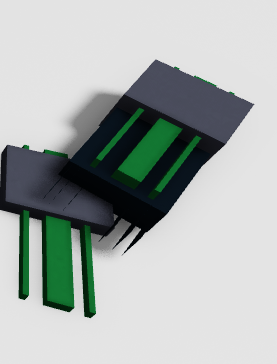# Unable to rotate / change orientation of the model with an script

I’m making a simulator game, and i wanna put a model into a specific CFrame position. That worked!

But its displaced, and i wanna rotate it by an script, once you press place it is there, but not rotated.

ONCE PRESSED: The card goes to the location but displaced:

This is how i would like it

The problem is, i dont know how to change the rotation of the model how do you change rotation of a model?

After i clicked the button i tried this:

``````	local mod = game.Workspace.icardx350:Clone()
mod:MakeJoints()
mod.Parent = workspace
mod:PivotTo(CFrame.new(-60.265, -51.309, 263.029))
``````

But rotation? Idk how?

Could someone help? Thanks!

Goodbye!

Try add this `mod:PivotTo(CFrame.angles(0, 0, 0--add the right orientation here))` i dont know if this works for sure though.

Uhh i tried everything thats possible, uhh… somethings wrong…`mod:PivotTo(CFrame.new(-60.265, -51.309, 263.029) * CFrame.Angles(math.rad(--[[rotation in degrees here]]),math.rad(--[[rotation in degrees here]]),math.rad(--[[rotation in degrees here]]))`
``````mod:PivotTo(CFrame.new(-60.265, -51.309, 263.029) * CFrame.Angles(math.rad(0), math.rad(10), math.rad(0)))